# Ringed space

(diff) ← Older revision | Latest revision (diff) | Newer revision → (diff)

A topological spacewith a sheaf of rings. The sheaf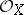is called the structure sheaf of the ringed space. It is usually understood thatis a sheaf of associative and commutative rings with a unit element. A pair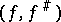is called a morphism from a ringed spaceinto a ringed space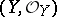ifis a continuous mapping and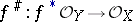is a homomorphism of sheaves of rings overwhich transfers units in the stalks to units. Ringed spaces and their morphisms constitute a category. Giving a homomorphismis equivalent to giving a homomorphismwhich transfers unit elements to unit elements.

A ringed space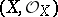is called a local ringed space ifis a sheaf of local rings (cf. Local ring). In defining a morphismbetween local ringed spaces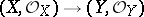it is further assumed that for any, the homomorphism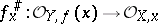is local. Local ringed spaces form a subcategory in the category of all ringed spaces. Another important subcategory is that of ringed spaces over a (fixed) field, i.e. ringed spaceswhereis a sheaf of algebras over, while the morphisms are compatible with the structure of the algebras.

### Examples of ringed spaces.

1) For each topological spacethere is a corresponding ringed space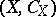, where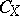is the sheaf of germs of continuous functions on.

2) For each differentiable manifold(e.g. of class) there is a corresponding ringed space, whereis the sheaf of germs of functions of class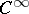on; moreover, the category of differentiable manifolds is a full subcategory of the category of ringed spaces over.

3) The analytic manifolds (cf. Analytic manifold) and analytic spaces (cf. Analytic space) over a fieldconstitute full subcategories of the category of ringed spaces over.

4) Schemes (cf. Scheme) constitute a full subcategory of the category of local ringed spaces.